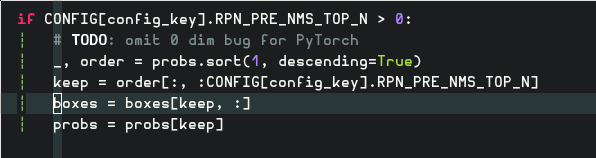# How to advancing like this?

For example, I use numpy:

Then I would like to do it in PyTorch, but it failed, I do not know how to solve it.

I know this is duplicated with How to get a matrix top-n in its second axis? However, `topk` still does not meet my real need that I wand to use `b` to index another variable`c` too. For example:@tom Hello, could you answer this question too? thank you!

My advand indexing in numpy is wrong too, forget it.

That is a weird thing to do. Did you intend to extract smallest 2 element for each row? If so, use this one liner

``````a.topk(2, dim=1, largest=False)
``````

if you don’t need result to be sorted, you can add `sorted=False` (defaulted to `True`).

What I want is below:

``````if CONFIG[config_key].RPN_PRE_NMS_TOP_N > 0:
# TODO: omit 0 dim bug for PyTorch
_, order = probs.sort(1, descending=True)
keep = order[:, :CONFIG[config_key].RPN_PRE_NMS_TOP_N]
index = torch.arange(0, boxes.size()).unsqueeze(1).long().cuda()
boxes = boxes[index, keep, :]
probs = probs[index, keep]
``````

So it is solved, but thank you anyway! @SimonW